• Have any questions?
• +91-9540-660064
• hahassignment@gmail.com

# Online Chemical Equilibrium Assignment Help

In a chemistry, Chemical Equilibrium is the distribution of interverting chemical compounds. More formally, equilibrium is the state in which the chemical activities or concentrations of the reactants and products have no net change over time. Usually, this state results when the forward chemical process proceeds at the same rate as their reverse reaction. The reaction rates of the forward and reverse reactions are generally not zero but, being equal, there are no net changes in the concentrations of the reactant and product. This process is called dynamic equilibrium.

### The chemical equilibrium has the following characteristics:

• Chemical equilibrium is dynamic in nature. The constancy of observable property in an equilibrium system does not mean that the reaction has stopped altogether. After the attainment of equilibrium the reaction does not stop although, it appears to have been stopped. The rates of two opposing reactions become equal. This means that if products are formed from the reactants, exactly equivalent amount of reactants being formed from the products.

For example, for the reaction between H2 and I2 to form HI,at equilibrium if certain amount of hydrogen iodide is formed by the combination of hydrogen and iodine, then exactly same amount of hydrogen iodide gets decomposed to give back H2 and I2

.

###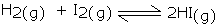The rate of formation of HI at the equilibrium becomes equal to the rate of decomposition of HI keeping the concentrations of the reactants and products constant. Since both the opposing reactions continue (at the same rate), even after the equilibrium, this chemical equilibrium is known as dynamic equilibrium.

• The properties of the system become constant at equilibrium and remain unchanged with time so long as the external factors remain the same. Fox example, in the decomposition of CaCO3 and evaporation of water in closed vessel, pressure becomes constant at equilibrium.
• The equilibrium can be attained only if the system is a closed one. If the system is not closed, some of the products may escape from the container and therefore, the backward reaction will not take place. Consequently, it will not be possible to attain the equilibrium.
• The equilibrium can be approached from either direction. For example, the equilibrium between nitrogen tetraoxide N2O4 (colourless gas) and nitrogen dioxide, NO2 (a reddish brown gas).

###It is observed that at ordinary temperature, say 298 K the two gases exist as an equilibrium mixture having pale brown colour. At very low temperature, (273 K) N2O4 is stable and exists as colourless gas. When the temperature is increased (373 K), it decomposes to NO2, which is dark brown in colour.

###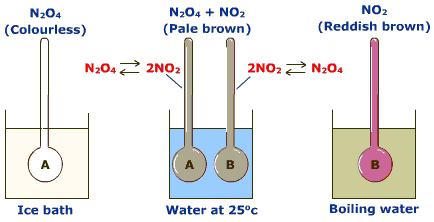Equilibrium between N2O4 and NO2

A catalyst does not alter the equilibrium point. In a reversible reaction, a catalyst increases the rate of forward as well as backward reaction to equal extent. Therefore, the equilibrium point is not altered. However, the equilibrium may be attained faster in the presence of a catalyst.

### Dynamic Nature of Chemical Equilibrium

The state of equilibrium is a state in which the measurable properties of the system do not undergo any noticeable change under a particular set of conditions.

We have seen that all observable properties of a system become constant at equilibrium. It may lead us to think that the reaction stops altogether at equilibrium. But this is not true. Actually at equilibrium the rate of forward reaction becomes equal to the rate of backward reaction so that there is no net change in the concentration of various species. In other words we can say that equilibrium state is a dynamic balance between the forward and backward reaction. This can be illustrated by considering the reaction between hydrogen and iodine to form hydrogen iodide.

###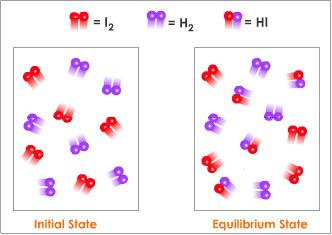When hydrogen and iodine are taken in a closed vessel maintained at 717k hydrogen molecules combine with iodine to form hydrogen iodide.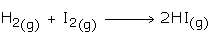The molecules of HI formed also begin to dissociate to form H2 and I2.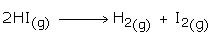###As the reaction progresses, the concentration of H2 and I2 decrease and hence rate of forward reaction slows down. On the other hand, concentration of hydrogen iodide increases and therefore the rate of backward reaction increases. A stage is reached when the rate of backward reaction becomes equal to the rate of forward reaction and the system attains equilibrium. The variation of the rate of forward reaction and backward reaction are shown in the graph. After this no change in concentration occurs provided the temperature of the reaction mixture is kept constant. Thus at equilibrium the reaction does not stop but the system acquires constant observable properties because of the equal rates of forward and backward reactions. Thus the equilibrium is dynamic in nature. The above reaction in equilibrium may be represented by the following reversible equation:The dynamic nature of chemical equilibrium can be demonstrated by adding small amount of radioactive iodine (I2*) to the above reaction in the state of equilibrium. It is noticed after some time that a mixture contains radioactive hydrogen iodide (HI*). It indicates that the reaction is going on even at equilibrium.indicates that the sample is radioactive.

The equilibrium achieved in physical processes such as dissolution of salt, evaporation of water etc. is called physical equilibrium whereas the equilibrium achieved in chemical processes such as reaction between H2 and I2 is called chemical equilibrium.

### Main Characteristics of Chemical Equilibrium

• The equilibrium can be attained only if the system is closed.
• A catalyst does not alter the equilibrium point.
• The equilibrium can be approached from either direction.
• The observable properties such as concentration, density of colour of the system become constant at equilibrium and remain unchanged thereafter.

### Dissociation of Electrolytes

Sodium chloride an ionic compound has the sodium ion, Na+ and chloride ion Cl- held together by strong electrostatic force of attraction in the solid state. When dissolved in water, the ions become free to move.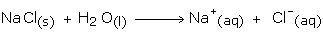The process of breaking of NaCl into free Na+ and Cl- ions is dissociation. NaCl is completely dissociated into Na+ and Cl- and is called a strong electrolyte.

Similarly a polar covalent compound like HCl is also dissociated into H+ and Cl- ions when added to water. Thus dilute hydrochloric acid too is a strong electrolyte.

Substances like CH3COOH which when added to water, dissociate partially as shown below: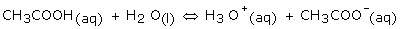In this case, all the molecules of CH3COOH do not dissociate into H3O+ and CH3COO- ions. There is equilibrium between the ions formed and the undissociated molecules. Such substances are called weak electrolytes. Other weak electrolytes are NH4OH, oxalic acid etc.

In case you face any problem or have any query please email us at :-info@homeworkassignmenthelp.com

### Submit us an Assignment:

Our tutors start working only after the payment is made, to ensure that we are doing work only for serious clients and also our solution meets the required standard.

### Getting homework help was never so easy you just need to follow following steps:

• Send us you Other Assignment or problem through email
• Specify the required format such as Word, Excel, Notepad, PDF
• Give us a deadline when you need the assignment completed along with the Time Zone.
(for example: EST, Australian GMT etc)
• Send documents related to your assignment which can help our tutors to provide a better work,
any example or format you want the solutions to be in.
• Our tutors will review the assignment sent by you and if all the required information is there we will
send you the price quoted by our tutor along with the time needed to solve the assignment
• You can pay us through paypal or credit card.
• After receiving the payment tutors start working on your assignment.
• Finally, we deliver the solutions and get a feedback from you regarding our work

In case you face any problem or have any query please email us at :- info@homeworkassignmenthelp.com